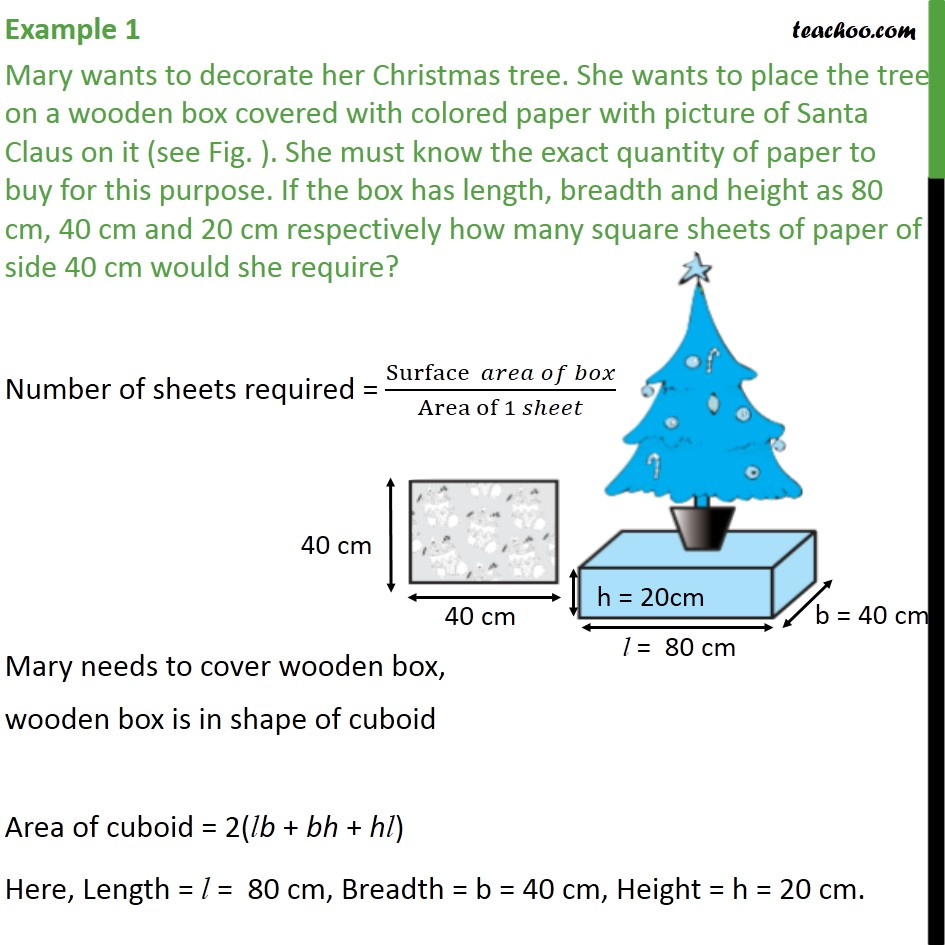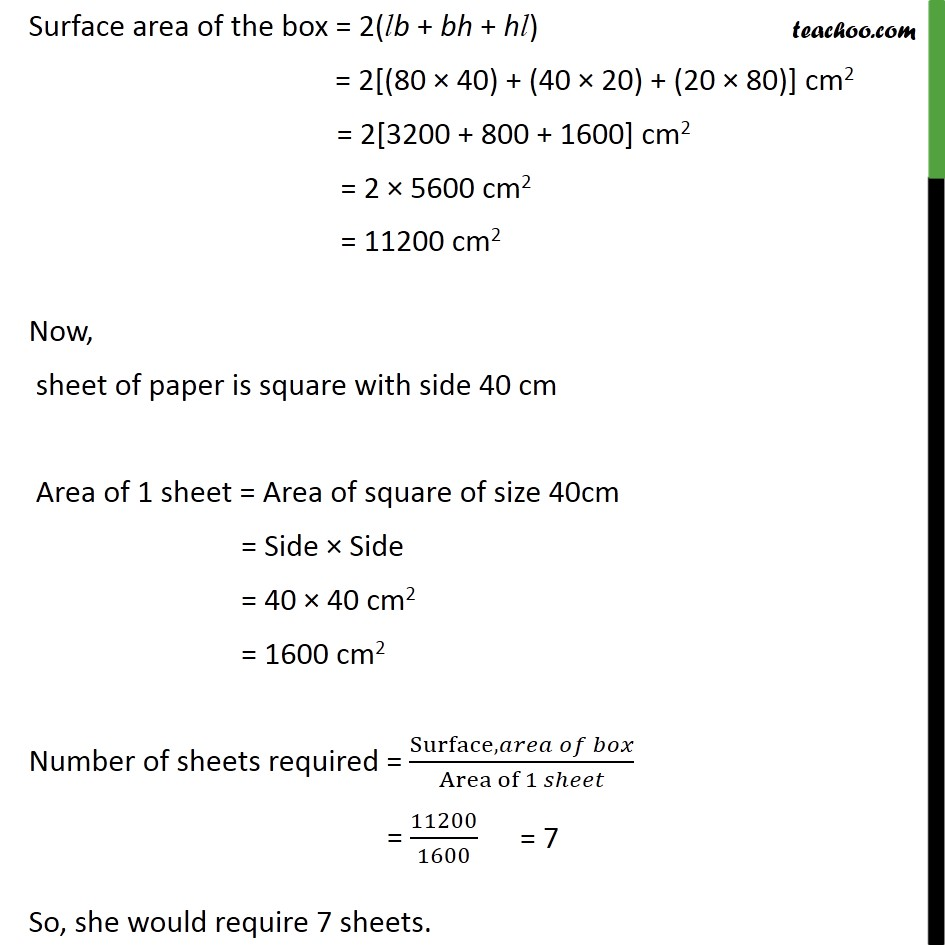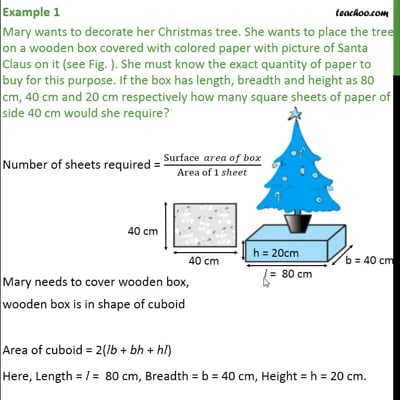Examples

Chapter 13 Class 9 Surface Areas and Volumes
Serial order wiseThis video is only available for Teachoo black users

Maths Crash Course - Live lectures + all videos + Real time Doubt solving!

### Transcript

Example 1 Mary wants to decorate her Christmas tree. She wants to place the tree on a wooden box covered with colored paper with picture of Santa Claus on it (see Fig. ). She must know the exact quantity of paper to buy for this purpose. If the box has length, breadth and height as 80 cm, 40 cm and 20 cm respectively how many square sheets of paper of side 40 cm would she require? Number of sheets required = (Surface )/(Area of 1 ) Mary needs to cover wooden box, wooden box is in shape of cuboid Area of cuboid = 2(lb + bh + hl) Here, Length = l = 80 cm, Breadth = b = 40 cm, Height = h = 20 cm. Surface area of the box = 2(lb + bh + hl) = 2[(80 40) + (40 20) + (20 80)] cm2 = 2[3200 + 800 + 1600] cm2 = 2 5600 cm2 = 11200 cm2 Now, sheet of paper is square with side 40 cm Area of 1 sheet = Area of square of size 40cm = Side Side = 40 40 cm2 = 1600 cm2 Number of sheets required = (Surface, )/(Area of 1 ) = 11200/1600 So, she would require 7 sheets.